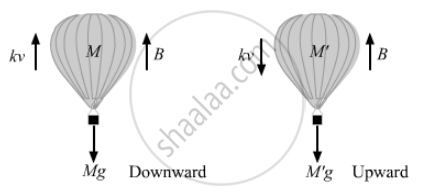# The Force of Buoyancy Exerted by the Atmosphere on a Balloon is B in the Upward Direction and Remains Constant. the Force of Air Resistance on the Balloon - Physics

Sum

The force of buoyancy exerted by the atmosphere on a balloon is B in the upward direction and remains constant. The force of air resistance on the balloon acts opposite the direction of velocity and is proportional to it. The balloon carries a mass M and is found to fall to the earth's surface with a constant velocity v. How much mass should be removed from the balloon so that it may rise with a constant velocity v?

#### Solution

Let M be mass of the balloon.
Let the air resistance force on balloon be F .
Given that F ∝ v.
⇒ F = kv,
where k = proportionality constant.When the balloon is moving downward with constant velocity,
B + kv = Mg    ...(i)
$\Rightarrow M = \frac{B + kv}{g}$
Let the mass of the balloon be M' so that it can rise  with a constant velocity v in the upward direction.
B = Mg + kv
$\Rightarrow M' = \frac{B + kv}{g}$
∴ Amount of mass that should be removed = M − M'.
$∆ M = \frac{B + kv}{g} - \frac{B - kv}{g}$
$= \frac{B + kv - B + kv}{g}$
$= \frac{2kv}{g} = \frac{2\left( Mg - B \right)}{g}$
$= 2\left\{ M - \frac{B}{g} \right\}$

Concept: Newton’s Second Law of Motion
Is there an error in this question or solution?

#### APPEARS IN

HC Verma Class 11, Class 12 Concepts of Physics Vol. 1
Chapter 5 Newton's Laws of Motion
Q 19 | Page 80